# Linux nl Command Tutorial for Beginners (7 Examples)

Linux offers a lot of text formatting command line tools, with each of them having a different purpose. One such utility is nl, which lets you number lines in files. In this tutorial, we will discuss the basics of this command using some easy to understand examples.

But before we do that, it's worth mentioning that all examples used in this tutorial have been tested on an Ubuntu 16.04 LTS system.

## Linux nl command

As already mentioned in the beginning, the nl command numbers lines in files. Following is its syntax:

`nl [OPTION]... [FILE]...`

And here's how the man page explains it:

`Write each FILE to standard output, with line numbers added. With no FILE, or when FILE is -, read standard input.`

Following are some Q&A styled examples that should give you a better idea on how nl works.

## Q1. How to use nl command?

Basic usage of nl is very easy - all you have to do is to pass as argument the name of the file whose lines you want to number.

`nl [filename]`

Here's an example: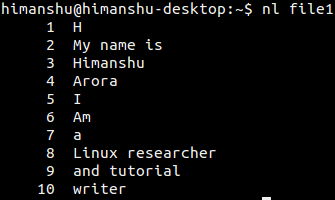## Q2. How to number empty lines?

By default, the nl command doesn't number empty lines: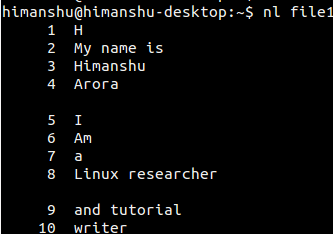However, if you want, you can change this behavior by passing value 'a' to the -b command line option.

`nl -b a [filename]`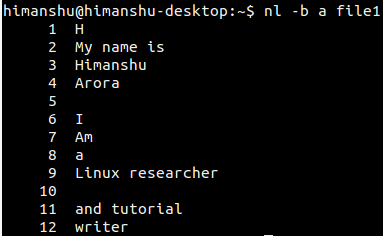## Q3. How to customize the number increment value?

By default, the number increment value is 1. However, you can customize this using the -i command line option.

`nl -i [new-inc-val] [filename]`

For example: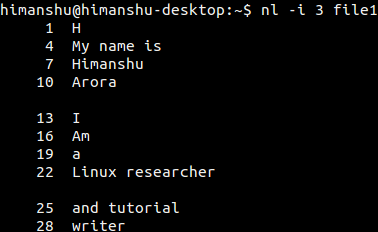## Q4. How to make nl consider multiple empty lines as one?

For this, use the -l command line option. Here's how the man page explains it:

` Consider NUMBER (default 1) consecutive empty lines to be one logical line for numbering, and only number the last one. Where fewer than NUMBER consecutive empty lines occur, do not number them. An empty line is one that contains no characters, not even spaces or tabs.`

For example, let's take the following file: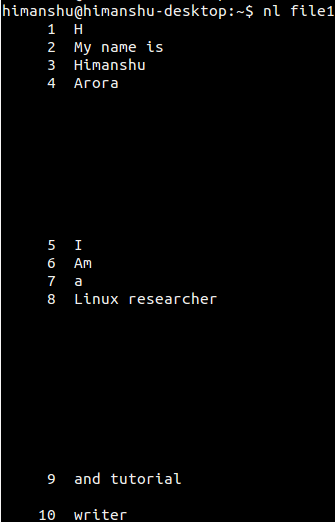And suppose, we want nl to consider 8 consecutive empty lines to be one logical line for numbering. Then here's the command we'd run:

`nl -b a -l 8 [filename]`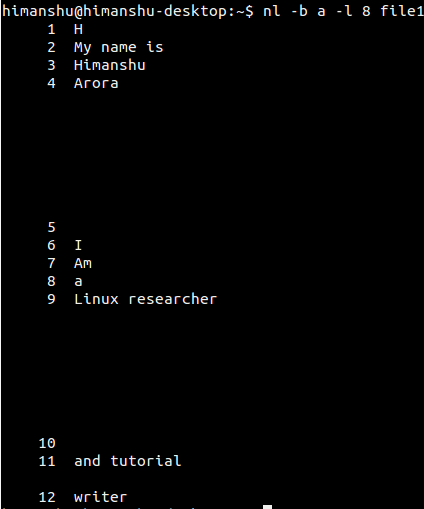## Q5. How to use different numbering formats?

You can use the -n command line option to specify numbering formats. Available option include left justified, right justified, and right justified with leading zeros. You can access these using the ln, rn, and rz values.

Here's an examples showing all these formats: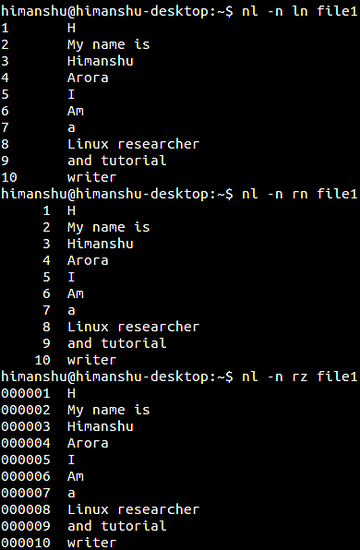## Q6.How to use a customized numbering separator?

By default, the character that separates number from the line is a TAB. However, if you want, you can customize this using the -s command line option, which requires the new separator as input.

For example, if we want to use a double colon (::) as the new separator, then here's how we can do that:

`nl -s :: [filename]`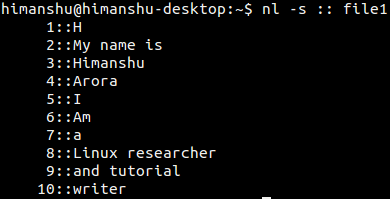## Q7. How to make nl use a different starting line number?

By default, numbering starts with 1. However, this you can change using the -v command line option. Here's how:

`nl -v [new-start-number] [filename]`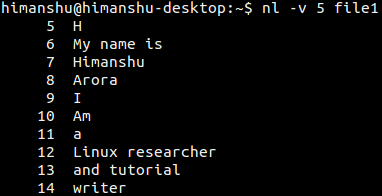## Conclusion

The nl command is easy to understand and use. Here, in this tutorial, we've covered majority of the command line options it offers. Once you are done practicing these, head to the tool's man page to learn more.view as pdf |print